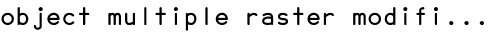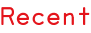RSS
object multiple raster modification
From:  jarrick
Date:  7. February 2011, 00:07

hi, I don't know anything about scripting as I am just starting to learn. I am running illustrator on windows.
I would like to modify the script that comes with scriptographer (object mulitple raster) so that does not take your objects and clone them randomly. I would like them to be cloned in the order that they are stacked in the layers pallet or something like that.
I tried taking out the line: "var item = dots[Math.floor(dots.length * Math.random())].clone();" but then the script didn't run.

// Pick a dot at random:
var item = dots[Math.floor(dots.length * Math.random())].clone();
item.position += new Point(x, y) * values.size;
item.rotate(radius * values.rotation * Math.PI / 180.0, item.position);
item.scale((radius * radius * values.gradiation + radius * (1.0 - values.gradiation)) * values.scale);
return item;

If you could help me with this, I will be most grateful,
thanks

Re: object multiple raster modification
Date:  7. February 2011, 12:37

Hi,

first off, declare an item counter like this

```var itemCounter = 0;
```

at the second row, then just exchange the line

```var item = dots[Math.floor(dots.length * Math.random())].clone();
```

with this one.

```var item = dots[itemCounter % dots.length].clone();
itemCounter++
```

Good luck!

Re: object multiple raster modification
From:  jarrick
Date:  7. February 2011, 16:04

I tried as you suggested so the script looks like this:

function createDot(x, y, dots, radius) {
if (radius > 0) {
// Pick a dot at random:
var itemCounter = 0;
var item = dots[itemCounter % dots.length].clone();
itemCounter++
item.position += new Point(x, y) * values.size;
item.rotate(radius * values.rotation * Math.PI / 180.0, item.position);
item.scale((radius * radius * values.gradiation + radius * (1.0 - values.gradiation)) * values.scale);
return item;
}
}

but it only creates the rasta half-tone image with the first object.
If I change
var itemCounter = 0;
to
var itemCounter = 1;
then it will create the half-tone image with the next object down so I understand that I am trying to increase that number for each clone. Is the code: itemCounter++ meant to increase that number by 1?

thanks

Re: object multiple raster modification
Date:  7. February 2011, 16:47

Sorry, I was a bit unclear about the counter.

The line

```var itemCounter = 0;
```

cannot be inside the createDot-function as it will be set as 0 (zero) EVERY time that particular function is called (ie. once per object) therefore the chosen object will always be item.

This should work better:

```var itemCounter = 0;

function createDot(x, y, dots, radius) {
if (radius > 0) {
var item = dots[itemCounter % dots.length].clone();
itemCounter++
item.position += new Point(x, y) * values.size;
item.rotate(radius * values.rotation * Math.PI / 180.0, item.position);
item.scale((radius * radius * values.gradiation + radius * (1.0 - values.gradiation)) * values.scale);
return item;
}
}
```

This way the itemCounter is set as zero only once (at the start of running the script), and is then increased by one with the line:

```itemCounter++
```

Hope that helps!

Re: object multiple raster modification
Date:  7. February 2011, 21:12

That works perfectly - thanks very much. I'm going to go through the tutorials again and see if I can wrap my head around it.

Scripts
08.08.14, 15:24
15.05.14, 14:23
02.03.14, 19:16
18.11.13, 14:48
22.03.13, 03:05
22.02.13, 15:45
Posts
10.01.17, 16:37
19.02.16, 06:03
19.02.16, 06:00
17.01.16, 11:00
12.01.16, 13:10
25.11.15, 08:19
Script of the Moment